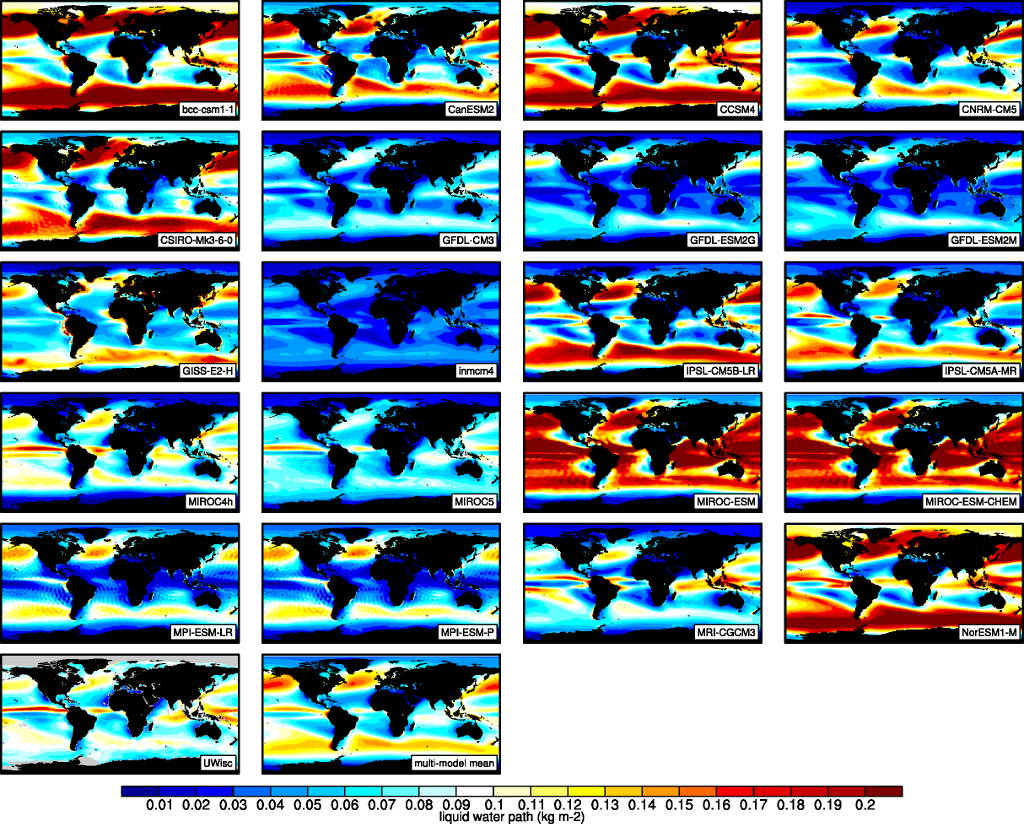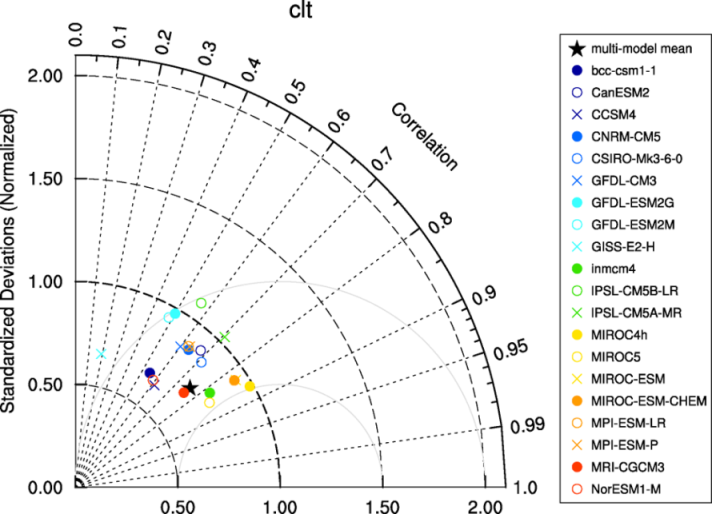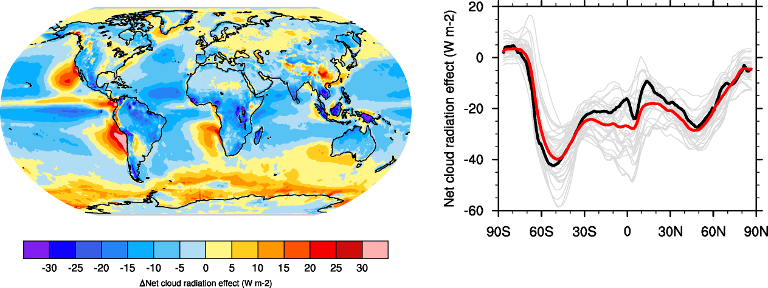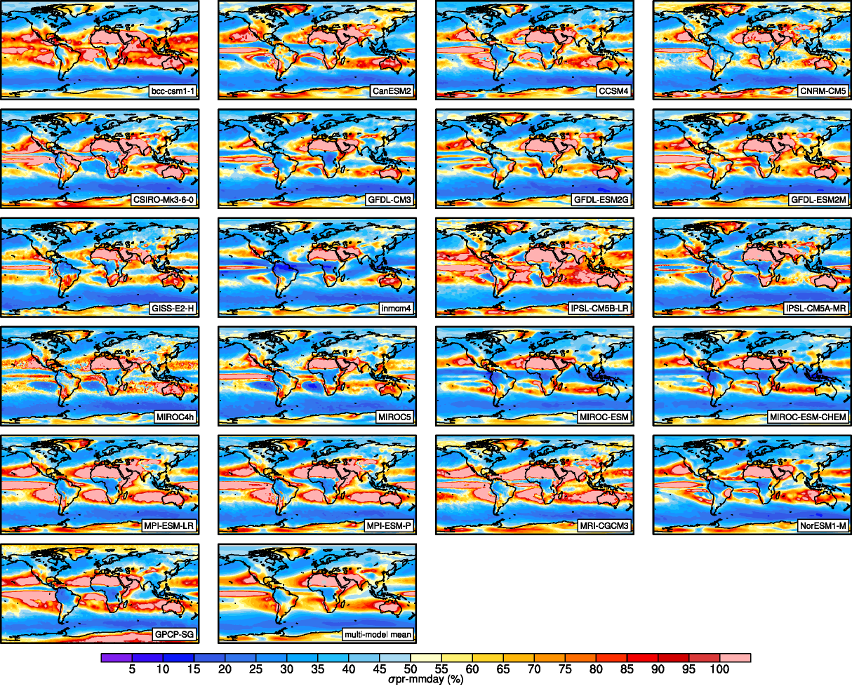# Clouds

## Overview

The recipe recipe_lauer13jclim.yml computes the climatology and interannual variability of climate relevant cloud variables such as cloud radiative forcing (CRE), liquid water path (lwp), cloud amount (clt), and total precipitation (pr) reproducing some of the evaluation results of Lauer and Hamilton (2013). The recipe includes a comparison of the geographical distribution of multi-year average cloud parameters from individual models and the multi-model mean with satellite observations. Taylor diagrams are generated that show the multi-year annual or seasonal average performance of individual models and the multi-model mean in reproducing satellite observations. The diagnostic also facilitates the assessment of the bias of the multi-model mean and zonal averages of individual models compared with satellite observations. Interannual variability is estimated as the relative temporal standard deviation from multi-year timeseries of data with the temporal standard deviations calculated from monthly anomalies after subtracting the climatological mean seasonal cycle.

## Available recipes and diagnostics

Recipes are stored in recipes/

• recipe_lauer13jclim.yml

Diagnostics are stored in diag_scripts/clouds/

• clouds.ncl: global maps of (multi-year) annual means including multi-model mean

• clouds_bias.ncl: global maps of the multi-model mean and the multi-model mean bias

• clouds_interannual: global maps of the interannual variability

• clouds_isccp: global maps of multi-model mean minus observations + zonal averages of individual models, multi-model mean and observations

• clouds_taylor.ncl: taylor diagrams

## User settings in recipe

1. Script clouds.ncl

Required settings (scripts)

none

Optional settings (scripts)

• embracesetup: true = 2 plots per line, false = 4 plots per line (default)

• explicit_cn_levels: explicit contour levels (array)

• extralegend: plot legend(s) to extra file(s)

• panel_labels: label individual panels (true, false)

• PanelTop: manual override for “@gnsPanelTop” used by panel plot(s)

• projection: map projection for plotting (default = “CylindricalEquidistant”)

• showdiff: calculate and plot differences model - reference (default = false)

• rel_diff: if showdiff = true, then plot relative differences (%) (default = False)

• ref_diff_min: lower cutoff value in case of calculating relative differences (in units of input variable)

• region: show only selected geographic region given as latmin, latmax, lonmin, lonmax

• timemean: time averaging - “seasonal” = DJF, MAM, JJA, SON), “annual” = annual mean

• treat_var_as_error: treat variable as error when averaging (true, false); true: avg = sqrt(mean(var*var)), false: avg = mean(var)

Required settings (variables)

none

• Optional settings (variables)

• long_name: variable description

• reference_dataset: reference dataset; REQUIRED when calculating differences (showdiff = True)

• units: variable units (for labeling plot only)

Color tables

• variable “lwp”: diag_scripts/shared/plot/rgb/qcm3.rgb

2. Script clouds_bias.ncl

Required settings (scripts)

none

Optional settings (scripts)

• plot_abs_diff: additionally also plot absolute differences (true, false)

• plot_rel_diff: additionally also plot relative differences (true, false)

• projection: map projection, e.g., Mollweide, Mercator

• timemean: time averaging, i.e. “seasonalclim” (DJF, MAM, JJA, SON), “annualclim” (annual mean)

• Required settings (variables)*

• reference_dataset: name of reference datatset

Optional settings (variables)

• long_name: description of variable

Color tables

• variable “tas”: diag_scripts/shared/plot/rgb/ipcc-tas.rgb, diag_scripts/shared/plot/rgb/ipcc-tas-delta.rgb

• variable “pr-mmday”: diag_scripts/shared/plots/rgb/ipcc-precip.rgb, diag_scripts/shared/plot/rgb/ipcc-precip-delta.rgb

3. Script clouds_interannual.ncl

Required settings (scripts)

none

Optional settings (scripts)

• colormap: e.g., WhiteBlueGreenYellowRed, rainbow

• explicit_cn_levels: use these contour levels for plotting

• extrafiles: write plots for individual models to separate files (true, false)

• projection: map projection, e.g., Mollweide, Mercator

Required settings (variables)

none

Optional settings (variables)

• long_name: description of variable

• reference_dataset: name of reference datatset

Color tables

• variable “lwp”: diag_scripts/shared/plots/rgb/qcm3.rgb

1. Script clouds_ipcc.ncl

Required settings (scripts)

none

Optional settings (scripts)

• explicit_cn_levels: contour levels

• mask_ts_sea_ice: true = mask T < 272 K as sea ice (only for variable “ts”); false = no additional grid cells masked for variable “ts”

• projection: map projection, e.g., Mollweide, Mercator

• styleset: style set for zonal mean plot (“CMIP5”, “DEFAULT”)

• timemean: time averaging, i.e. “seasonalclim” (DJF, MAM, JJA, SON), “annualclim” (annual mean)

• valid_fraction: used for creating sea ice mask (mask_ts_sea_ice = true): fraction of valid time steps required to mask grid cell as valid data

Required settings (variables)

• reference_dataset: name of reference data set

Optional settings (variables)

• long_name: description of variable

• units: variable units

Color tables

• variables “pr”, “pr-mmday”: diag_scripts/shared/plot/rgb/ipcc-precip-delta.rgb

2. Script clouds_taylor.ncl

Required settings (scripts)

none

Optional settings (scripts)

• embracelegend: false (default) = include legend in plot, max. 2 columns with dataset names in legend; true = write extra file with legend, max. 7 dataset names per column in legend, alternative observational dataset(s) will be plotted as a red star and labeled “altern. ref. dataset” in legend (only if dataset is of class “OBS”)

• estimate_obs_uncertainty: true = estimate observational uncertainties from mean values (assuming fractions of obs. RMSE from documentation of the obs data); only available for “CERES-EBAF”, “MODIS”, “MODIS-L3”; false = do not estimate obs. uncertainties from mean values

• filename_add: legacy feature: arbitrary string to be added to all filenames of plots and netcdf output produced (default = “”)

• mask_ts_sea_ice: true = mask T < 272 K as sea ice (only for variable “ts”); false = no additional grid cells masked for variable “ts”

• styleset: “CMIP5”, “DEFAULT” (if not set, clouds_taylor.ncl will create a color table and symbols for plotting)

• timemean: time averaging; annualclim (default) = 1 plot annual mean; seasonalclim = 4 plots (DJF, MAM, JJA, SON)

• valid_fraction: used for creating sea ice mask (mask_ts_sea_ice = true): fraction of valid time steps required to mask grid cell as valid data

Required settings (variables)

• reference_dataset: name of reference data set

Optional settings (variables)

none

## Variables

• clwvi (atmos, monthly mean, longitude latitude time)

• clivi (atmos, monthly mean, longitude latitude time)

• clt (atmos, monthly mean, longitude latitude time)

• pr (atmos, monthly mean, longitude latitude time)

• rlut, rlutcs (atmos, monthly mean, longitude latitude time)

• rsut, rsutcs (atmos, monthly mean, longitude latitude time)

## Observations and reformat scripts

Note: (1) obs4MIPs data can be used directly without any preprocessing; (2) use `esmvaltool data info DATASET` or see headers of reformat scripts for non-obs4MIPs data for download instructions.

• CERES-EBAF (obs4MIPs) - CERES TOA radiation fluxes (used for calculation of cloud forcing)

• GPCP-SG (obs4MIPs) - Global Precipitation Climatology Project total precipitation

• MODIS (obs4MIPs) - MODIS total cloud fraction

• UWisc - University of Wisconsin-Madison liquid water path climatology, based on satellite observbations from TMI, SSM/I, and AMSR-E, reference: O’Dell et al. (2008), J. Clim.

Reformat script: esmvaltool/cmorizers/data/formatters/datasets/uwisc.ncl

## Example plotsFig. 4 The 20-yr average LWP (1986-2005) from the CMIP5 historical model runs and the multi-model mean in comparison with the UWisc satellite climatology (1988-2007) based on SSM/I, TMI, and AMSR-E (O’Dell et al. 2008).Fig. 5 Taylor diagram showing the 20-yr annual average performance of CMIP5 models for total cloud fraction as compared to MODIS satellite observations.Fig. 6 20-year average (1986-2005) annual mean cloud radiative effects of CMIP5 models against the CERES EBAF (2001–2012). Top row shows the shortwave effect; middle row the longwave effect, and bottom row the net effect. Multi-model mean biases against CERES EBAF are shown on the left, whereas the right panels show zonal averages from CERES EBAF (thick black), the individual CMIP5 models (thin gray lines) and the multi-model mean (thick red line). Similar to Figure 9.5 of Flato et al. (2013).Fig. 7 Interannual variability of modeled and observed (GPCP) precipitation rates estimated as relative temporal standard deviation from 20 years (1986-2005) of data. The temporal standard devitions are calculated from monthly anomalies after subtracting the climatological mean seasonal cycle.